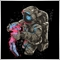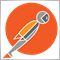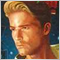95

Hi guys,

I have been struggling adding a feature to my EA.

I want to opposite open market orders on every trade at my SL level that get deleted once TP is hit.

So basically, if I have a long position and the SL is hit, I want to go short at the same level as my SL. If TP is hit, the order gets deleted.

At the moment, the EA opens long position is the H4 MACD is positive and the H1 MACD is negative and the H1 RSI is oversold. Vice versa for short positions. SL and TP are set as well.

Thanks a lot!
Martin

```//+------------------------------------------------------------------+

input double PositionSize                       = 1;      // Position size (cost of one pip)
input int H4short                               = 21;     // H4 MACD
input int H4long                                = 480;    // H4 MACD
input int H4med                                 = 3;      // H4 MACD
input int H1short                               = 21;     // H1 MACD
input int H1long                                = 48;     // H1 MACD
input int H1med                                 = 3;      // H1 MACD
input int rsi_ma_period                         = 14;     // RSI
input int StopLossDistance                      = 50;     // Stop loss distance in pips
input bool IsUseTrailingStop                    = false;  // Use trailing stop
input int  TrailingStopDistance                 = 40;     // Trailing stop distance in pips
input bool IsUseCrossStochasticLinesCondition   = false;  // Use condition "%K line crosses the %D line"
input bool UseTakeProfit                        = false;  // Use Take Profit
input int  Take_Profit                          = 50;     // Take Profit
input int RSIuplimit                            = 70;     // RSI Upper Limit
input int RSIlowlimit                           = 30;     // RSI Lower Limit

double Res;
int Previouscount;
int    rsi_handle=0;
bool   ext_hedging=true;
CSymbolInfo symbol_info;
ulong arr_ticket_long_rsi[];
ulong arr_ticket_short_rsi[];
double trailing_stop_value;
double stop_loss_value;
double take_profit_value;
int tick_counter=0;
int time_in_open_function=-1;
int time_in_close_function=-1;
double M15shortData[], M15longData[], M15medData[], H4shortData[], H4longData[], H4medData[], H1shortData[], H1longData[], H1medData[]; // You can declare multiple variables of the same data type in the same line.
int numberofM15Data, numberofH4Data, numberofH1Data;
int M15ControlPanel, H4ControlPanel, H1ControlPanel;
double M15MACD1, M15MACD2, H4MACD1, H4MACD2, H1MACD1, H1MACD2;

#define MA_MAGIC 2719281928459450

//+------------------------------------------------------------------+
//| Expert initialization function                                   |
//+------------------------------------------------------------------+
int OnInit()
{

H4ControlPanel = iMACD(_Symbol, PERIOD_H4, H4short, H4long, H4med, PRICE_CLOSE);
H1ControlPanel = iMACD(_Symbol, PERIOD_H1, H1short, H1long, H1med, PRICE_CLOSE);

//   shortemaControlPanel = iMACD(_Symbol, _Period, shortemaPeriods, longemaPeriods, medemaPeriods, PRICE_CLOSE);

//--- prepare trade class to control positions if hedging mode is active
ext_hedging=((ENUM_ACCOUNT_MARGIN_MODE)AccountInfoInteger(ACCOUNT_MARGIN_MODE)==ACCOUNT_MARGIN_MODE_RETAIL_HEDGING);
symbol_info.Name(_Symbol);

if(symbol_info.Digits()==3 || symbol_info.Digits()==5)

rsi_handle=iRSI(_Symbol, PERIOD_H1,rsi_ma_period,PRICE_CLOSE);
if(rsi_handle==INVALID_HANDLE)
{
printf("Error creating RSI oscillator");
return(INIT_FAILED);
}

ArrayResize(arr_ticket_long_rsi,0,100000);
ArrayResize(arr_ticket_short_rsi,0,100000);

//-----
return(INIT_SUCCEEDED);
}
//+------------------------------------------------------------------+
//| Expert deinitialization function                                 |
//+------------------------------------------------------------------+
void OnDeinit(const int reason)
{

}

//+------------------------------------------------------------------+
//| Expert tick function                                             |
//+------------------------------------------------------------------+
void OnTick()
{

numberofH4Data = CopyBuffer(H4ControlPanel,0,0,3,H4shortData);
numberofH1Data = CopyBuffer(H1ControlPanel,0,0,3,H1shortData);

H4MACD1 = H4shortData;
H4MACD2 = H4shortData;
H1MACD1 = H1shortData;
H1MACD2 = H1shortData;

currentBid = SymbolInfoDouble(_Symbol,SYMBOL_BID); // Get latest Bid Price

//CheckForClose();
CheckForOpen();

}

{
double Profit;
int MagicNumber;
{
Res = 0;

if (HistorySelect(0, INT_MAX))
for (int i = HistoryDealsTotal() - 1; i >= 0; i--)
{
const ulong Ticket = HistoryDealGetTicket(i);

if((HistoryDealGetInteger(Ticket, DEAL_MAGIC) == MagicNumber) && (HistoryDealGetString(Ticket, DEAL_SYMBOL) == Symbol()))
Res += HistoryDealGetDouble(Ticket, DEAL_PROFIT);
}
Print(Res);
//   return(Res);
}
}

//+------------------------------------------------------------------+
//| Try to open the deal                                          |
//+------------------------------------------------------------------+
void CheckForOpen()

{

MqlRates rt;
if(CopyRates(_Symbol,_Period,0,2,rt)!=2)
{
Print("CopyRates of ",_Symbol," failed, no history");
return ;
}

datetime cur_time=rt.time;
MqlDateTime mdt;
TimeToStruct(cur_time,mdt);
int cur_calculated_time=mdt.hour*60+mdt.min;
if(cur_calculated_time==time_in_open_function)
return;
time_in_open_function=cur_calculated_time;
/*if(rt.tick_volume>1)
return ;*/

//remove unnecessary orders
RefreshOrders();
//move trailing stop orders if it is necessary
ModifyOrders();

//reserve array for RSI
double   rsi;

//get RSI values
if(CopyBuffer(rsi_handle,0,0,3,rsi)!=3)
{
Print("CopyBuffer from iRSI failed, no data");
return ;
}

string str_date_time= TimeToString(TimeCurrent())+" ";

//check condition "Stochastic indicator (%K line) leaves the 0-20 zone"
//to open long position
//-----

if(H4MACD1 > 0)
{
if(H1MACD1 < 0)
{

//check condition "RSI indicator leaves the 0-30 zone"
//to open long position
if(rsi<RSIlowlimit && rsi>=RSIlowlimit)
{
Alert("Open long position, because RSI leaves 0;30 zone");
Print("Try to open long for ",_Symbol);
return;
}

if (Res < 0)
{
OpenPosition(arr_ticket_short_rsi,ORDER_TYPE_SELL);
}

}
}

//to open short position
//-----

if(H4MACD1 < 0)
{
if(H1MACD1 > 0)
{
//check condition "RSI indicator leaves the 70-100 zone"
//to open short position
if(rsi>RSIuplimit && rsi<=RSIuplimit)
{
Alert("Open short position, because RSI leaves 70-100 zone");
Print("Try to open short for ",_Symbol);
OpenPosition(arr_ticket_short_rsi,ORDER_TYPE_SELL);
}

if (Res < 0)
{
}

}
}
}
//+------------------------------------------------------------------+
//| Open position                                                  |
//+------------------------------------------------------------------+
void OpenPosition(ulong& arr_ticket[],ENUM_ORDER_TYPE order_type)
{

{
string str_date_time= TimeToString(TimeCurrent())+" ";
double stop_loss=0;
double take_profit=0;
double local_stop_loss_value=IsUseTrailingStop ? trailing_stop_value:stop_loss_value;

//        double local_take_profit_value= UseTakeProfit ? take_profit_value:take_profit_value;
double bid_price=SymbolInfoDouble(_Symbol,SYMBOL_BID);
//Calculate stop loss price
if(local_stop_loss_value!=0)
NormalizeDouble(bid_price-local_stop_loss_value,Digits());

// Calculating take profit price
//        if(local_take_profit_value!=0)
take_profit=order_type==ORDER_TYPE_SELL ? NormalizeDouble(bid_price-take_profit_value,Digits()):

//                                                       NormalizeDouble(bid_price+local_take_profit_value,Digits());

//Calculate lot size
double lot_size=GetLotSize();
string str_comment=_Symbol+_Period+TimeToString(TimeCurrent());
stop_loss,take_profit,str_comment);
if(is_open)
{
Print("Terminal opened the deal for ",_Symbol);
uint total=PositionsTotal();
for(uint i=0; i<total; i++)
{
string symbol=PositionGetSymbol(i);
string comment=PositionGetString(POSITION_COMMENT);
if(symbol==_Symbol && comment==str_comment)
{
ticket=PositionGetInteger(POSITION_TICKET);

}

}
int array_size=ArraySize(arr_ticket);
ArrayResize(arr_ticket,array_size+1);
arr_ticket[array_size]=ticket;

}
else
{
Print("Terminal could not open deal for ",_Symbol);
Print("Terminal error code= ",result_ret_code);
}
}

}

//+------------------------------------------------------------------+
//| Close position with the specified ticket                                                 |
//+------------------------------------------------------------------+
void ClosePosition(ulong& arr_tickets[])
{
string date_time_string=TimeToString(TimeCurrent())+" ";
for(int i=0;i<ArraySize(arr_tickets);i++)
{
ulong ticket=arr_tickets[i];

bool is_selected=PositionSelectByTicket(ticket);
if(is_selected)
{

{
if(!is_close)
{
Print("Terminal could not close the position for",_Symbol," the error Code=",result_ret_code);
}
}

}
else
{

}
ticket=0;

}
ArrayResize(arr_tickets,0,10000);
}
//+------------------------------------------------------------------+
//| Remove unnecessary orders from tickets array                                                 |
//+------------------------------------------------------------------+
void RefreshArray(ulong& arr_ticket[])
{
ulong buffer_array[];
ArrayResize(buffer_array,0,10000);
int count=0;
for(int i=0;i<ArraySize(arr_ticket);i++)
{
ulong ticket=arr_ticket[i];
bool is_selected=PositionSelectByTicket(ticket);
if(is_selected)
{
ArrayResize(buffer_array,count+1,10000);
buffer_array[count]=ticket;
count++;
}
}
if(ArraySize(buffer_array)!=ArraySize(arr_ticket))
{
ArrayResize(arr_ticket,ArraySize(buffer_array),10000);
ArrayCopy(arr_ticket,buffer_array,0,0,WHOLE_ARRAY);
}
}
//+------------------------------------------------------------------+
//| Remove unnecessary orders from all tickets array                                                 |
//+------------------------------------------------------------------+
void RefreshOrders()
{
RefreshArray(arr_ticket_long_rsi);
RefreshArray(arr_ticket_short_rsi);
}
//+------------------------------------------------------------------+
//| Get lot size for the deal                                                 |
//+------------------------------------------------------------------+
double GetLotSize()
{
double lot_step=SymbolInfoDouble(_Symbol,SYMBOL_VOLUME_STEP);
double min_lot=SymbolInfoDouble(_Symbol,SYMBOL_VOLUME_MIN);
double max_lot=SymbolInfoDouble(_Symbol,SYMBOL_VOLUME_MAX);
lot_size_for_deal=GetLotSize(lot_size_for_deal, lot_step,min_lot,max_lot);
return lot_size_for_deal;
}
//+------------------------------------------------------------------+
//| Get best fit lot size for the calculated one
//+------------------------------------------------------------------+
double GetLotSize(double calculated_lot_size,double lot_size_step,double min_lot_size,double max_lot_size)
{
if(calculated_lot_size<min_lot_size)
return min_lot_size;
if(calculated_lot_size>max_lot_size)
return max_lot_size;
double cur_lot_size=lot_size_step;
double next_lot_size=cur_lot_size+lot_size_step;
do
{
if(calculated_lot_size>=cur_lot_size && calculated_lot_size<=next_lot_size)
{
if(calculated_lot_size==cur_lot_size)
return cur_lot_size;
if(calculated_lot_size==next_lot_size)
return next_lot_size;
if(calculated_lot_size-cur_lot_size<next_lot_size-calculated_lot_size)
return cur_lot_size;
else
return next_lot_size;
}
cur_lot_size=next_lot_size;
next_lot_size=cur_lot_size+lot_size_step;
}while(true);
}
//+------------------------------------------------------------------+
//| Move trailing stop if it is necessary for all orders                                                 |
//+------------------------------------------------------------------+
void ModifyOrders()
{
ModifyOrders(arr_ticket_long_rsi);
ModifyOrders(arr_ticket_short_rsi);
}
//+------------------------------------------------------------------+
//| Move trailing stop if it is necessary for all orders from array                                                |
//+------------------------------------------------------------------+
void ModifyOrders(ulong& arr_ticket[])
{

if(!IsUseTrailingStop || TrailingStopDistance==0)
return;
for(int i=0;i<ArraySize(arr_ticket);i++)
{
ulong ticket=arr_ticket[i];
bool is_selected=PositionSelectByTicket(ticket);
if(is_selected)
{
ENUM_POSITION_TYPE position_type=PositionGetInteger(POSITION_TYPE);
double open_price=PositionGetDouble(POSITION_PRICE_OPEN);
double current_stop_loss=PositionGetDouble(POSITION_SL);
double local_stop_loss;
double bid_price=SymbolInfoDouble(_Symbol,SYMBOL_BID);
{
if(bid_price-open_price>trailing_stop_value)
{
local_stop_loss=NormalizeDouble(bid_price-trailing_stop_value,Digits());
if(current_stop_loss<local_stop_loss)
{
}
}
}
else if(position_type==POSITION_TYPE_SELL)
{
{
if(current_stop_loss>local_stop_loss)
{
}

}
}

}
}

}
```2380

Here's how you do it the easy way which may require you to think differently about orders than you're used to. There is no such thing as a "Stop loss order". A stop-loss is a command send along with the market order instructing your broker to set a stop-order at the same time it executes your market position. You can have the same net result by opening a market position and sending a separate stop-order with equal volume as the market order. So when you say you want to reverse at a stoploss, what you really need is a stop-order that is twice the volume of your market order. When the price hits the stop order the original position will be closed and you will have an opposite position of equal volume (reversed by stop).

Here is a MVE snippet to try it for yourself.

```#include <trade/trade.mqh>

void OnStart()
{
CAccountInfo acc;
return;
}

{
CSymbolInfo symbol;
symbol.Name(_Symbol);
double sl = symbol.NormalizePrice(
open_price - sl_points * symbol.Point()
);
}
return false;
}

```95

nicholi shen:

Here's how you do it the easy way which may require you to think differently about orders than you're used to. There is no such thing as a "Stop loss order". A stop-loss is a command send along with the market order instructing your broker to set a stop-order at the same time it executes your market position. You can have the same net result by opening a market position and sending a separate stop-order with equal volume as the market order. So when you say you want to reverse at a stoploss, what you really need is a stop-order that is twice the volume of your market order. When the price hits the stop order the original position will be closed and you will have an opposite position of equal volume (reversed by stop).

Here is a MVE snippet to try it for yourself.

Thank you very much for the quick reply, I highly appreciate it.

Where should the bool marketBuyWithReversingStop be place? In OnTick?

I will put this in my code and let you know how I get on!376

//+------------------------------------------------------------------+
//+------------------------------------------------------------------+
{
//--- get transaction type as enumeration value
//--- if transaction is result of addition of the transaction in history

{
long     deal_entry        =0;
long     deal_type         =0;
double   deal_price        =0.0;
double   deal_profit       =0.0;
double   deal_volume       =0.0;
string   deal_symbol       ="";
long     deal_magic        =0;
int      deal_reason       =0;
if(HistoryDealSelect(trans.deal))
{
deal_entry=HistoryDealGetInteger(trans.deal,DEAL_ENTRY);
deal_type=HistoryDealGetInteger(trans.deal,DEAL_TYPE);
deal_price=HistoryDealGetDouble(trans.deal,DEAL_PRICE);
deal_profit=HistoryDealGetDouble(trans.deal,DEAL_PROFIT);
deal_volume=HistoryDealGetDouble(trans.deal,DEAL_VOLUME);
deal_symbol=HistoryDealGetString(trans.deal,DEAL_SYMBOL);
deal_magic=HistoryDealGetInteger(trans.deal,DEAL_MAGIC);
deal_reason=HistoryDealGetInteger(trans.deal,DEAL_REASON);
}
else
return;

if (deal_entry==DEAL_ENTRY_OUT && deal_reason==DEAL_REASON_SL)
{
}

}
}2380

Martin_2017:

Thank you very much for the quick reply, I highly appreciate it.

Where should the bool marketBuyWithReversingStop be place? In OnTick?

I will put this in my code and let you know how I get on!

I'm sorry but this isn't like you're decorating a webpage with a CSS snippet. You can't just plop it into your code and expect it to work. It's your responsibility to understand the logic and how implement it yourself.95

nicholi shen:
I'm sorry but this isn't like you're decorating a webpage with a CSS snippet. You can't just plop it into your code and expect it to work. It's your responsibility to understand the logic and how implement it yourself.

Alright, no need to be defensive and snob about it, it was only a question.

Thanks for the help anyway, I do appreciate it.95

Enrique Dangeroux:
//+------------------------------------------------------------------+
//+------------------------------------------------------------------+
{
//--- get transaction type as enumeration value
//--- if transaction is result of addition of the transaction in history

{
long     deal_entry        =0;
long     deal_type         =0;
double   deal_price        =0.0;
double   deal_profit       =0.0;
double   deal_volume       =0.0;
string   deal_symbol       ="";
long     deal_magic        =0;
int      deal_reason       =0;
if(HistoryDealSelect(trans.deal))
{
deal_entry=HistoryDealGetInteger(trans.deal,DEAL_ENTRY);
deal_type=HistoryDealGetInteger(trans.deal,DEAL_TYPE);
deal_price=HistoryDealGetDouble(trans.deal,DEAL_PRICE);
deal_profit=HistoryDealGetDouble(trans.deal,DEAL_PROFIT);
deal_volume=HistoryDealGetDouble(trans.deal,DEAL_VOLUME);
deal_symbol=HistoryDealGetString(trans.deal,DEAL_SYMBOL);
deal_magic=HistoryDealGetInteger(trans.deal,DEAL_MAGIC);
deal_reason=HistoryDealGetInteger(trans.deal,DEAL_REASON);
}
else
return;

if (deal_entry==DEAL_ENTRY_OUT && deal_reason==DEAL_REASON_SL)
{